## Cho m gam FeO tác dụng hết với dung dịch H2SO4, thu được 200 ml dung dịch FeSO4 1M. Giá trị của m là

Question

Cho m gam FeO tác dụng hết với dung dịch H2SO4, thu được 200 ml dung dịch FeSO4 1M. Giá trị của m là

in progress 0
6 months 2021-07-17T07:34:46+00:00 1 Answers 1438 views 0

1. Answer: The mass of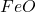required is 14.37 g

Explanation:

Molarity is calculated by using the equation: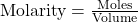……(1)

We are given:

Molarity of iron (II) sulfate = 1 M

Volume of solution = 200 mL = 0.200 L (Conversion factor: 1 L = 1000 mL)

Putting values in equation 1, we get: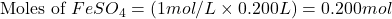The chemical equation for the reaction of FeO with sulfuric acid follows: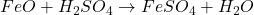By stoichiometry of the reaction:

If 1 mole of iron (II) sulfate is produced by 1 mole of FeO

So, 0.200 moles of iron (II) sulfate will produce =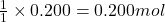of FeO

The number of moles is defined as the ratio of the mass of a substance to its molar mass. The equation used is: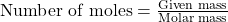We know, molar mass of= 71.84 g/mol

Putting values in above equation, we get: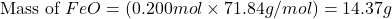Hence, the mass ofrequired is 14.37 g